# Carmichael number facts for kids

Kids Encyclopedia Facts

In number theory a Carmichael number is a composite positive integer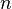$n$, which satisfies the congruence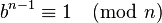$b^{n-1}\equiv 1\pmod{n}$ for all integers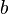$b$ which are relatively prime to$n$. Being relatively prime means that they do not have common divisors, other than 1. Such numbers are named after Robert Carmichael.

All prime numbers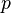$p$ satisfy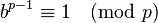$b^{p-1}\equiv 1\pmod{p}$ for all integers$b$ which are relatively prime to$p$. This has been proven by the famous mathematician Pierre de Fermat. In most cases if a number$n$ is composite, it does not satisfy this congruence equation. So, there exist not so many Carmichael numbers. We can say that Carmichael numbers are composite numbers that behave a little bit like they would be a prime number.

## Images for kidsCarmichael number Facts for Kids. Kiddle Encyclopedia.